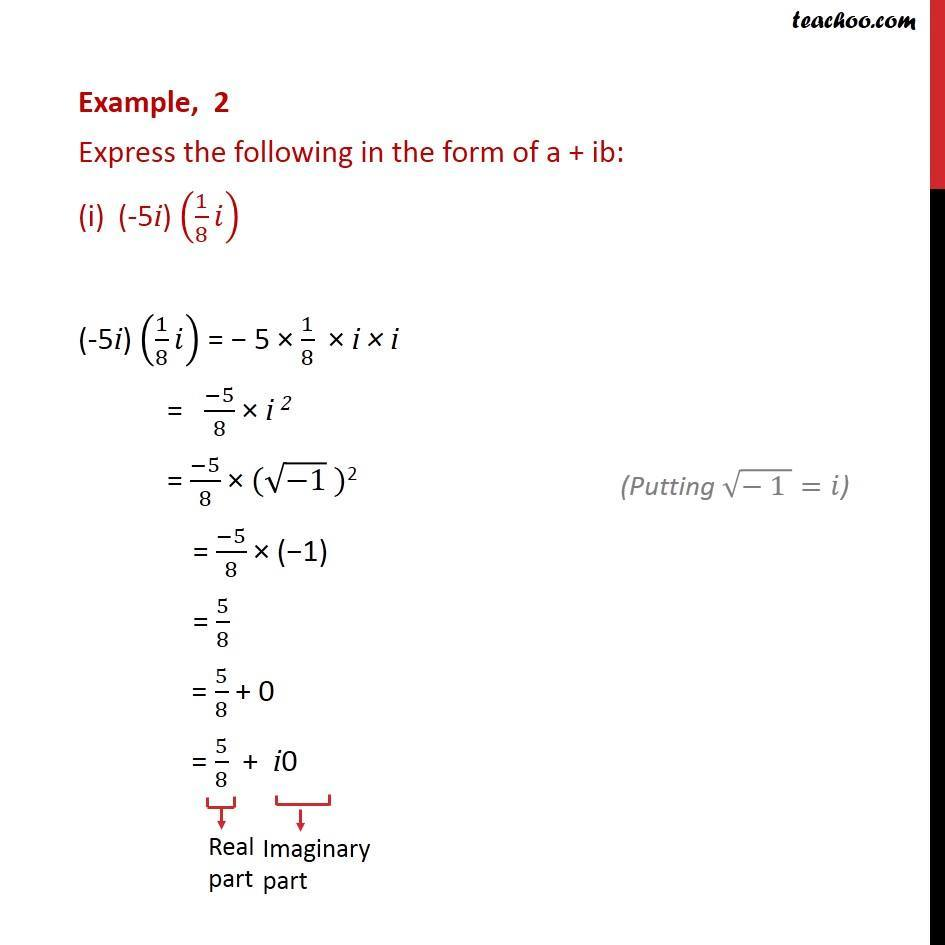Power of i(odd and even)

Chapter 5 Class 11 Complex Numbers (Term 1)
Concept wise### Transcript

Example, 2 Express the following in the form of a + ib: (-5i) (1/8 ) (-5i) (1/8 ) = 5 1/8 i i = ( 5)/8 i 2 = ( 5)/8 ( ( 1) )2 = ( 5)/8 ( 1) = 5/8 = 5/8 + 0 = 5/8 + i0